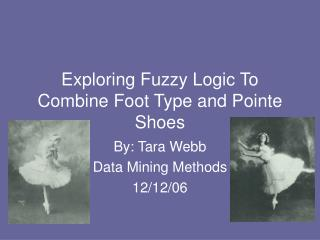DownloadDownload PresentationExploring Fuzzy Logic To Combine Foot Type and Pointe Shoes

# Exploring Fuzzy Logic To Combine Foot Type and Pointe Shoes

Télécharger la présentation## Exploring Fuzzy Logic To Combine Foot Type and Pointe Shoes

- - - - - - - - - - - - - - - - - - - - - - - - - - - E N D - - - - - - - - - - - - - - - - - - - - - - - - - - -
##### Presentation Transcript

1. Exploring Fuzzy Logic To Combine Foot Type and Pointe Shoes By: Tara Webb Data Mining Methods 12/12/06

2. Outline • What are Pointe Shoes? How is foot type involved in making a decision on which kind to buy? • Confusion in Finding Appropriate Shoe • What is Fuzzy Logic? • How does a fuzzy system work? • How does it work mathematically? • Overview

3. Pointe Shoes • Special shoe used by female ballet dancers • Made of canvas, paper, and glue • Many different brands available • Two Important Features: • The box • The shank

4. Fitting Pointe Shoes Flat Feet Normal Feet High Arched Feet

5. Fuzzy Logic: Human Vs. Computer Thinking • Humans use fuzzy logic in their everyday lives! • Humans evaluate in a fuzzy manner. • Computers evaluate in precise values.

6. Fuzzy Logic System

7. Mathematically Speaking: • Foundations of Fuzzy Logic can be thought of as an extension of set theory • A set can be described as a membership function, mA(x), defined over some “Universe of Discourse.” • mA(x) = 1 when x is an element of the set • mA(x) = 0 when x is not an element

8. Mathematically Speaking Cont’d: • In a fuzzy set, mA(x) could be values other than 0 or 1. • This is a way of describing a percentage of how much the element belongs to the set. • This leads to the if-then statement: • IF A THEN B

9. Mathematically Speaking Cont’d: • The fuzzy sets of A and B are combined by using the Cartesian Product, R = A X B • This R takes on a membership equal to the min {mA(a), mB(b)} for each (a,b) pair • The results are then aggregated by taking the maximum membership for each state in the “then” part across all the results of each rule. • The last step is to “defuzzify” the aggregated set to get a crisp output value.

10. Overview • Fuzzy logic is based on the way humans evaluate. • It gives percentages of belonging which lead to a precise action. • This percentage of belonging directly relates to the problem of matching foot types to pointe shoes. • It is my recommendation that this would be an optimal way to solve this problem.

11. References: • Feet and Foot Types. Retrieved October 8, 2006, from www.dancer.com/foottype • Fuzzy Logic. Retrieved October 8, 2006 from http://en.wikipedia.org/wiki/Fuzzy_logic • Kaehler, Steven D. (n.d.). Fuzzy Logic Tutorial. Retrieved November 18, 2006, from • http://www.seattlerobotics.org/encoder/mar98/fuz/flindex.html • Micalewicz, Z. & Fogel, D. (2002). How to Solve It: Modern Heuristics. New York: Springer. • Pointe Shoes. Retrieved October 8, 2006, from http://en.wikipedia.org/wiki/Pointe_shoes • Sowell, Thomas. (n.d.). Fuzzy Logic Tutorial. Retrieved October 8, 2006, from http://www.fuzzy-logic.com • What is My Foot Type?. Retrieved October 8, 2006, from • www.northlan.gov.uk/leisure+and+tourism/sports+activities/running/what+is+my+foot+type